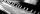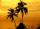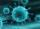# Loan

Apply for a \$ 59000 loan, the loan repayment period is 8 years, the interest rate 7%.
How much should I pay for every month (or every year if paid yearly).

Example is for practise geometric progression and/or periodic payment for an annuity.

Result

m =  804.39 USD
y =  9880.6 USD

#### Solution:Leave us a comment of example and its solution (i.e. if it is still somewhat unclear...):Be the first to comment!## Next similar examples:

1. InterestCalculate how much you earn for 10 years 43000 deposit if the interest rate is 1.3% and the interest period is a quarter.
2. Compound interestCompound interest: Clara deposited CZK 100,000 in the bank with an annual interest rate of 1.5%. Both money and interest remain deposited in the bank. How many CZK will be in the bank after 3 years?
3. DepositIf you deposit 959 euros the beginning of each year, how much money we have at 2.1% (compound) interest after 19 years?
4. BankPaul put 10000 in the bank for 6 years. Calculate how much you will have in the bank if he not pick earned interest or change deposit conditions. The annual interest rate is 3.5%, and the tax on interest is 10%.
5. PopulationWhat is the population of the city with 3% annual growth, if in 10 years the city will have 60,000 residents?
6. PianoIf Suzan practicing 10 minutes at Monday; every other day she wants to practice 2 times as much as the previous day, how many hours and minutes will have to practice on Friday?
7. One halfOne half of ? is: ?
8. Tenth memberCalculate the tenth member of geometric sequence when given: a1=1/2 and q=2
9. Geometric sequence 4It is given geometric sequence a3 = 7 and a12 = 3. Calculate s23 (= sum of the first 23 members of the sequence).
10. Eight palmThere grows 8 palms by the sea. At the first sitting one parrot, on second two, on third sits four parrots on each other twice the previous parrots sitting on a previous palm. How many parrots sitting on eighth palm?
11. Five membersWrite first 5 members geometric sequence and determine whether it is increasing or decreasing: a1 = 3 q = -2
12. Theorem proveWe want to prove the sentence: If the natural number n is divisible by six, then n is divisible by three. From what assumption we started?
13. Geometric progression 2There is geometric sequence with a1=5.7 and quotient q=-2.5. Calculate a17.
14. Six termsFind the first six terms of the sequence a1 = -3, an = 2 * an-1
15. VirusWe have a virus that lives one hour. Every half hour produce two child viruses. What will be the living population of the virus after 3.5 hours?
16. PowersExpress the expression ? as the n-th power of the base 10.
17. GP - 8 itemsDetermine the first eight members of a geometric progression if a9=512, q=2